### Index Formula: Understanding It in Excel With 10 Different Examples About It

An index is a function in the Microsoft Excel with two different forms of syntax: reference and array. When using it in the array form, then it will be returning with either a value or array of different values. Array form is useful when first argument is constant to array.

Index Formula and Parameters (Arguments)

The index function has different arguments or parameters as some people calls it. The complete formula is array, then row number (row_num), followed by column number (column_num), which is the last part of the index’s arguments.

Array: This is a range of either cells or constant array. If the array has one row/column, then a row number or column number that corresponds to it is optional. If an array has more than one row/column to used, and only either a column or row number is used, then the index will return in the array of a whole column or row that is in the array.

Row number (row_num): This will select a row that is in the array, from which it can return to with its value. If this is omitted, then it is critical to include the column number. It is important that the argument point to a cell that is inside the array, so it does not return with the #REF! value, which is an error.

Column number (column_num): The user has the opportunity to select a column that is in an array, from which it can return with a value. If this is omitted, then the row number is essential to the index function formula. This is an argument that must be pointing to a cell that is within an array, otherwise it will be returning with the #REF error value.

Example 1: Simple Index Formula

In this example, the business is going through some things, and we wants to evaluate how the business is doing, which is why we are looking for how many sales that someone has made for the period. This is where the index formula has proven to be useful in finding out the answer to the question, which is how many sales Frank Thompson has made.

=INDEX(A3:J10,2,6)Example 2: Formula with Named Table

This example focuses on using the table to find the answer, which means we have layout the data, and this has been given a name, which makes it necessary to use the text, and if you are having the same circumstances, this example would be useful for you.

=INDEX(Table1,4,7)Example 3: Index and Average Formula

This example is about our concerns with the products that are in the warehouse, which is why we need to know how our products are performing on an average level, and the problem needs to be assessed, because the products in warehouse are costing too much money in inventory, and they are all just lying there, we want to know the average of the products that are stored. This is where both the average and index formula would be extremely useful to get the desired answer.

=AVERAGE(INDEX(A3:J10,,7))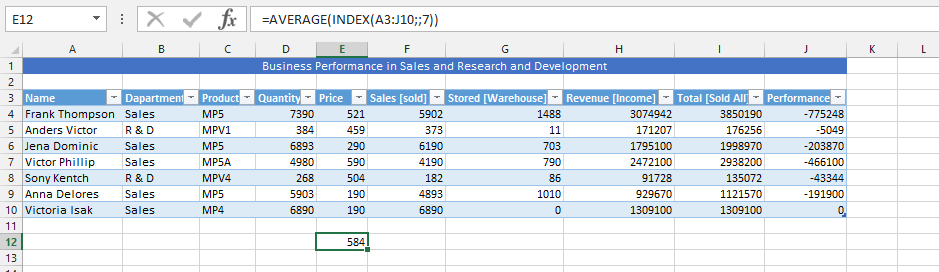Example 4: Using the Index and VLOOK Up to Find Answers

The business is going quite well, but we would like to know how it would be working out, especially since the stored products also worth some money, but we cannot find some answers that would identify how much the product worth, when the business is having some issues about its inventory. This is where we are going to use both index and Vlookup functions to work together for finding how much the inventory worth, if we had sold it.

=INDEX(A3:J10,4,7) * VLOOKUP(“Victor Phillip”,A3:J10,5,FALSE)Example 5: Sum & Index Formulas Simultaneously Use

This example focuses on finding the person who mostly performed in the business. We are looking for their performances in form of sales, earnings, and where their performances mostly increased. However, we have come to prefer finding out how many products are stored in warehouse, and find out how the business’ is performing when it comes to the quantity that has been stored in the warehouse. This is where sum and working together to find the answer that we are looking for, and finding the answer that is relevant to the information we are looking for.

=SUM(G4:INDEX(A3:J10,8,7))Example 6: COUNTA with INDEX Formula

We are using both the index and COUNTA formulas to work on a highly performing period, to know what it says, and how this is possibly achievable. This is where we will use both COUNTA and INDEX formula to find the answers that we are looking for.

=INDEX(A3:J10,COUNTA(“MP5”,F6),8)Example 7: Two Different Index Formula in One

In this example, we are going to use the index formula two times to multiply a specific value with another value, so we could find something that we are looking for, because the performance of business needs to be assessed in different ways, and with this formula, we will be able to make further assessments to the business overall performance.

=INDEX(A3:J10,2,7)*INDEX(A3:J10,2,5)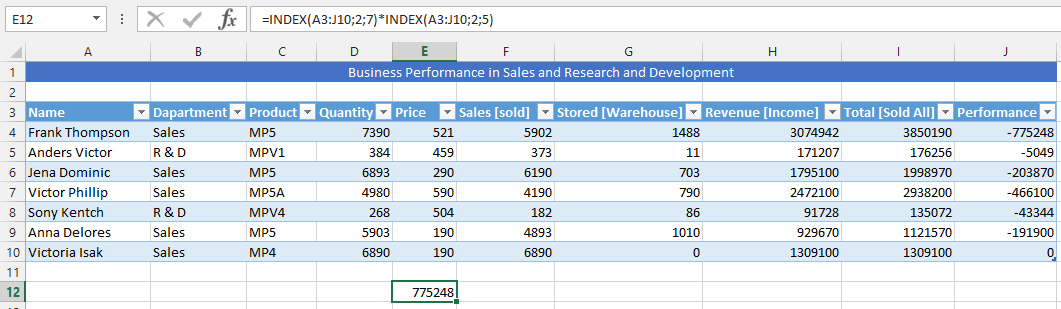Example 8: Triple Index Formula

This is a triple formula we are going to use under the circumstances that we would like to verify that the information we have provided to the Performance part of the whole table, and make it possible and easy to find out if the performance, which is actually to determine if we are on minus in financial performance or if we are on positive when the sales would be made. This would result in making it easier to assess how the business is performing.

=INDEX(A3:J10,2,7)*INDEX(A3:J10,2,5) & INDEX(A3:J10,2,10)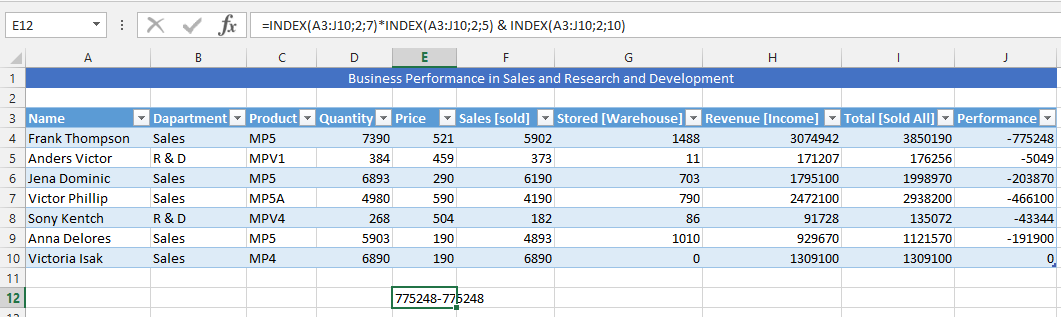Example 9: Both Index and Max Formula in One

In this example, we are looking for the performance of the market, and searching to acknowledge how much money the business is making at most in a specific area. This is where both the index and max formula would be very useful for acknowledging this answer.

=MAX(H4:H10 INDEX(A3:J10,,))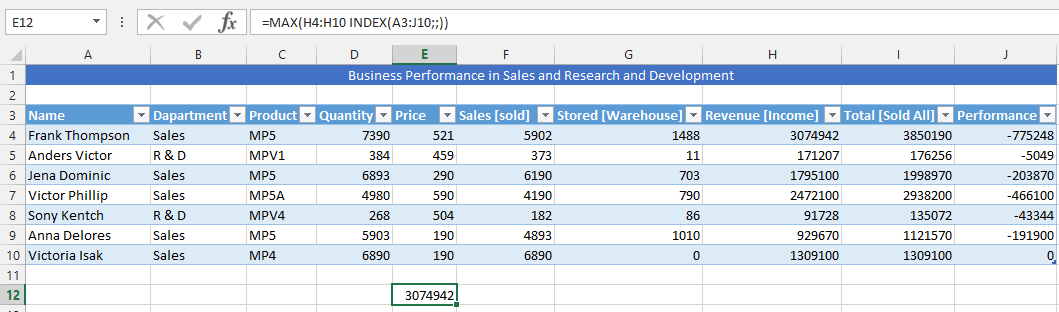Example 10: Min and Index Formula in One

The previous example has provided us with the opportunity of highest performance in the result, and we want to know the minimum performance of the company. This will allow the business to assess its strength and weakness. In this example both the minimum and index formula would be used for finding out how the business is performing in the long run.

=MIN(H4:H10 INDEX(A3:J10,,))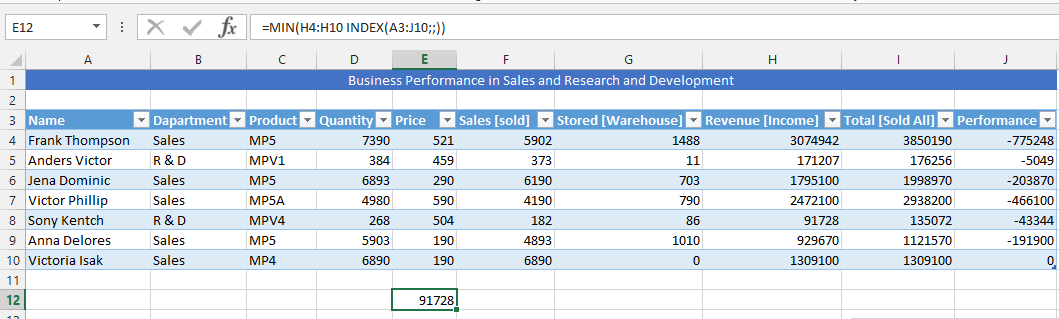## Template

```Further reading: